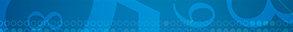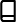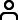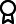Journal metrics
See full report
Acceptance rate17%
Submission to final decision55 days
Acceptance to publication40 days
CiteScore1.600
Journal Citation Indicator0.330
Impact Factor-

### Indexing news

International Journal of Mathematics and Mathematical Sciences has recently been accepted into Web of Science.

####Journal profile

International Journal of Mathematics and Mathematical Sciences publishes research across all fields of mathematics and mathematical sciences, such as pure and applied mathematics, mathematical physics, probability and mathematical statistics.

####Editor spotlight

International Journal of Mathematics and Mathematical Sciences maintains an Editorial Board of practicing researchers from around the world, to ensure manuscripts are handled by editors who are experts in the field of study.

####Special Issues

Do you think there is an emerging area of research that really needs to be highlighted? Or an existing research area that has been overlooked or would benefit from deeper investigation? Raise the profile of a research area by leading a Special Issue.

## Latest Articles

More articles
Research Article

### Connected -Chainable Sets and Existence Results

In the setting of -chainable metric spaces, we introduce uniformly local weak contraction and obtain some results on the existence of fixed points. To show the veracity of the results, we also constructed some examples.

Research Article

### Stability Conditions for a Nonlinear Time Series Model

This research aims to determine whether the proposed time series model is stable or not. To achieve this, Ozaki’s approximation method was utilized, where the nonlinear part is a function that approaches zero, similar to Ozaki’s imposed function. The study produced examples of both stable and unstable models, and it was discovered that the stability and instability of the model are affected by the enforced optional constants. The researchers used the approximation method to obtain an asymptotic linear model that satisfied the singular point of the proposed model. The study first identified the singular points of the suggested model and then focused on determining the stability conditions, which was the main objective of the study. Last, the stability conditions of the limit cycle were established.

Research Article

### The Intersection Multiplicity of Intersection Points over Algebraic Curves

In analytic geometry, Bézout’s theorem stated the number of intersection points of two algebraic curves and Fulton introduced the intersection multiplicity of two curves at some point in local case. It is meaningful to give the exact expression of the intersection multiplicity of two curves at some point. In this paper, we mainly express the intersection multiplicity of two curves at some point in and under fold point, where . First, we give a sufficient and necessary condition for the coincidence of the intersection multiplicity of two curves at some point and the smallest degree of the terms of these two curves in . Furthermore, we show that two different definitions of intersection multiplicity of two curves at a point in are equivalent and then give the exact expression of the intersection multiplicity of two curves at some point in under fold point.

Research Article

### Analysis of Turberculosis-COVID-19 Coinfection Using Fractional Derivatives

Fractional-order derivative modeling continues to receive great interest among researchers across the globe. In this study, Tuberculosis-COVID-19 coinfection is studied using Atangana–Baleanu fractional-order derivatives defined in Caputo sense. We confirmed the existence and singularity of the solution and investigated the model’s equilibrium points. Additionally, we examined the model’s stability in terms of the Ulam–Hyers and generalized Ulam–Hyers stability criteria. The basic reproduction number was calculated using the next-generation matrix approach. We also looked into the model’s disease-free equilibrium point’s regional stability. Numerical scheme for simulating the fractional-order system with Mittag–Leffler Kernels are presented. Numerical simulations are given to validate the model. Results of the simulation showed a decline in the number of COVID-19 infections within the population when the fractional operator was reduced.

Research Article

### Equivalence for Various Forms of Quadratic Functional Equations and Their Generalized Hyers–Ulam–Rassias Stability

In this manuscript, we study the properties and equivalence results for different forms of quadratic functional equations. Using the Brzdȩk and Ciepliński fixed-point approach, we investigate the generalized Hyers–Ulam–Rassias stability for the 3-variables quadratic functional equation in the setting of 2-Banach space. Also, we obtain some hyperstability results for the 3-variables quadratic functional equation. The results obtained in this paper extend several known results of the literature to the setting of 2-Banach space.

Research Article

### The Characteristic of the Minimal Ideals and the Minimal Generalized Ideals in Rings

In this paper, we prove that the characteristic of a minimal ideal and a minimal generalized ideal, which is meant to be one of minimal left ideal, minimal right ideal, bi-ideal, quasi-ideal, and -ideal in a ring, is either zero or a prime number . When the characteristic is zero, then the minimal ideal (minimal generalized ideal) as additive group is torsion-free, and when the characteristic is , then every element of its additive group has order . Furthermore, we give some properties for minimal ideals and for generalized ideals which depend on their characteristics.Journal metrics
See full report
Acceptance rate17%
Submission to final decision55 days
Acceptance to publication40 days
CiteScore1.600
Journal Citation Indicator0.330
Impact Factor-Author guidelinesEditorial boardDatabases and indexingAuthor guidelinesEditorial boardDatabases and indexing

Article of the Year Award: Outstanding research contributions of 2021, as selected by our Chief Editors. Read the winning articles.